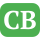CppBuzz.com

# Loops in C Programming

Loops allow to execute a block of code repeatedly until a certain condition is matched. Loop minmizes coding effort when we want to execute same block on code multiple times.

## Types of Loops

• for
• while
• do while()

All of these loops can be used in achieving the same task but there is difference among these

## for loop

Syntax :

```for(initialization; condition; increment/decrement)
{
//code
}
```

Other Possible Syntax 2:

```for(initialization; condition;)
{
//code
increment/decrement
}
```

Other Possible Syntax 3:

```initialization
for( ; condition; increment/decrement)
{
//code

}
```

Other Possible Syntax 4:

```initialization
for( ; condition; )
{
//code
increment/decrement

}
```

Points to remember about for loop

• for loop must has three semicolumn i.e ;
• We can also nested for loop
• We can have multiple initialization separated by comma - i=0, j=0, k=0
• for( ; ; ) is an infinite for loop
Example on for loop

```#include <stdio.h>

int main()
{
int i;
for(i = 0; i<6; i++)
{
printf("\n for loop in C");
}
return 0;
}
```

The above code will print "for loop in C" 6 times.

How does for loop work

Step 1. The initialization statement is executed only once.
Step 2. The condition is evaluated. If the condition is is true; the for loop's contents are executed. But if the condition is false then loop is terminated and loop's contents are not executed.
Step 3. After executing the loop's content, increment/decrement take place and then condition is checked again. Follow step 2 again.

Hence step 2 & step 3 repeated until loop is terminated.

## while loop

Compared to for loop the while loop has only condition. If the conditon is false then loop is terminated. And If the condition is true then contents of while is executed and condition is evaluated again.

Syntax :

```
while(condition)
{
//code
}
```
Example on for while loop

```#include <stdio.h>

int main()
{
int i = 0;
while( i<6 )
{
printf("\n while loop in C");
i++;
}
return 0;
}
```

The above code will print "while loop in C" 6 times.

Other Possible Syntax 2:

```#include <stdio.h>

int main()
{
int i = 0;
while( i++<6 )
{
printf("\n while loop in C");

}
return 0;
}
```

## do-while() loop

Syntax :

```do{
//code
}while(condition);
```

Example on do while

```#include <stdio.h>

int main()
{
int i=0;
do
{
printf("\n do-while() loop in C");
i++;
}while(i<6);
return 0;
}
</xmp</pre>

<p class="plabel"><strong>2nd Example on do while</strong></p>
<pre><xmp>#include <stdio.h>

int main()
{
int i=0;
do
{
printf("\n do-while() loop in C");

}while(i++<6);
return 0;
}
```

What is next? You may solve MCQs on loops in C++

MCQs on Loops in C++